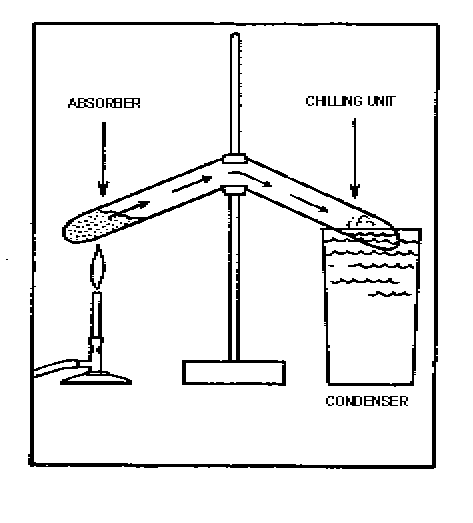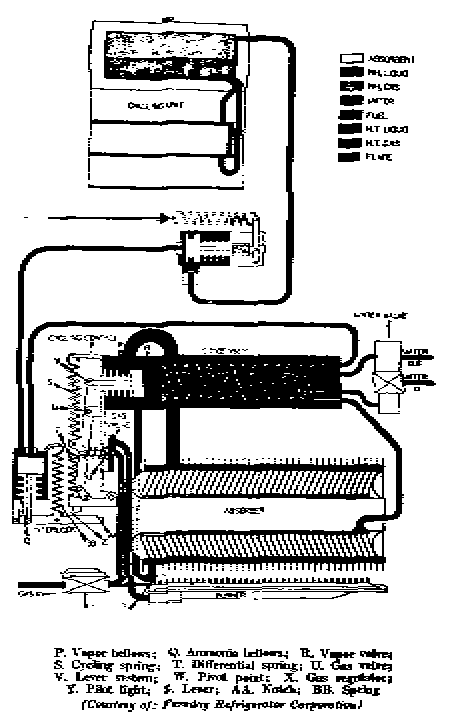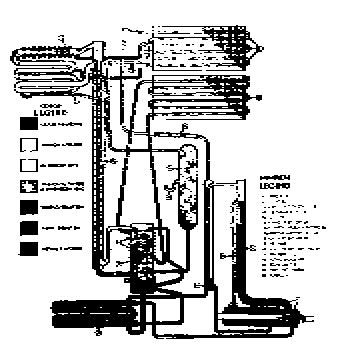# HEAT

The Atomic "Wiggle Wiggle" Dance.
The Atomic Dance That Keeps Us Warm.

In the ordinary sense, heat is the measure of vibration between atoms in a molecule. At absolute zero, there is no inter–atomic motion. No motion, no heat; Zero; Our " Reference Point "!   This is where we mathematically place our "Zero" [ 0 ].

Therefore, there is no such creature as cold. Cold is a slang term and should not be used in science. Something can be cool, which is a relative term, when referenced, but, Cold is not an Entity, like Heat is !

So, what about those other zeros [ 0's ] you have heard about ?

• Fahrenheit

Those are for the weather man. He could use the Kelvin scale, which uses centigrade divisions and starts at the " No Motion — Absolute Zero " point.

" Our over–night low temperature will be around 333 degrees. "
Which is 60 degrees on the regular Centigrade scale.   ( 273 + 60 = 333 )

Or, how about using the English Fahrenheit, scale divisions ?

" Our high tomorrow, will possibility reach the 550 degree mark. "
That's 90 degrees Fahrenheit.   ( 460 + 90 = 550 )

Temperature Conversions: – Enter a number in either field, then click outside the text box.
Temperature   F: C:

Most of you know that there is more to the Temperature Story than this, but, ...

let's move along with heat.

### Heat energy is expressed as Two components:

Sensible Heat and Latent Heat.

• SENSIBLE HEAT: That which can sensed by our bodies, and directly measured with a thermometer, using one of our three common scales.

• Kelvin
• Fahrenheit

So, Temperature can be defined as how fast the dance is going; the Tempo.

• LATENT HEAT: The additional heat required to change the state of a substance from solid to liquid at its melting point, or from liquid to gas at its boiling point, after the temperature of the substance has reached either of these points. This is often referred to as a substance's "Change of State" energy.

Ops, this quality doesn't equate directly to the thermometer !

So, Temperature Does Not tell you How Much Heat there is !

At any given temperature, a cubic centimeter of iron has more kinetic heat energy, than a cubic centimeter of Aluminum.

Different materials can hold different amounts of heat. The amount of heat that a material can hold is Specific for that material. We refer to it in the reference books as .... Yup .... " Specific Heat ".

Specific Heat is largely the result of Density. The number of dancers on the dance floor. You all know how Hot, a crowded dance floor can be.

When we want to know " How Much Heat ", we have, or need, we must consider the temperature in relation to " Specific Heat ", and weather-or-not there is any " Change of State Energy " involved.

{ Reference } X { Temperature } X { Specific Heat } X { Weight }
Plus
{ Change of State Energy }

" Temperature ", measured in degrees; multiplied by " Specific Heat ";
multiplied by " Weight ", measured in ...

Darn !   Here is where a nice simple concept like Heat, gets messed up.

 Which " Degrees " are we using for temperature ? Kelvin Centigrade Fahrenheit And, what kind of scale do we need for weight ? For our measurements, do we use ... Grams Kilograms Ounces Pounds Tons Metric Ton Troy Ounces Quintal Grains Pennyweight Scruples Carat Dram

Systems of Measurement
Needed to Engineer Atoms
*Units Used by Most Reference Books
Metric Vs English
HEAT — Calories   [ Cal. ]

Temperature — Degrees Kelvin

Weight — Grams

Length — Meters

Volume — Cubic Centimeters

Pressure — Dynes / cm2

*HEAT — British Thermo Units   [ Btu. ]

Temperature — Degrees Fahrenheit

Weight — Ounces; Pounds; Tons; ...

Length — Inches; Feet; Yards; ...

Volume — Ounces; Quarts; Gallons; ...

Pressure — Pounds / in2; Inches of Hg

( Just another little problem with our Scientific Language. )

That which is inappropriately called Radiant Heat is a sub-set of LIGHT.

It is possible to "Pump Heat" from one place to another, even if the destination is at a higher energy level than the source. This technology is derived from the Science of Thermodynamics.

Lucky for us, Mother Nature has established a consistent set of rules or laws that remain constant throughout the ages. Various people have discovered these "Laws" through observation and experimentation, and often these relationships are named after them.

## Boyle's Law

( for "perfect gases" )

"The volume of a gas varies inversely as the pressure, provided the temperature remains constant."

This means that, if a certain quantity of gas has its pressure doubled, the volume becomes one half of the original. Conversely, if the volume becomes doubled, the gas has its pressure reduced by one half.

This law is based on absolute temperature and absolute pressure.

Absolute   Zero =   – 460 degrees Fahrenheit or – 273 degrees Centigrade.

Absolute Pressure is based on the pressure of outer–space being equal to 0. The average "absolute pressure" at sea level is equal to 14.7 pounds per square inch.

## Dalton's Law

The total pressure of a mixture of gases, is the sum of the partial pressures of each of the gases in the mixture. The law further explains that each gas behaves as if it occupies the space alone. Also, according to this law, the temperature at which a liquid boils, or at which a gas condenses in an enclosed space corresponds to the partial pressure exerted by that gas.

Let me repeat! Each gas behaves as if, it were the ONLY gas in the room. This is a Very Important Point, as we will quickly experience, as we study how Water moves about in your "Tortoise Shell".

Substances always exert a pressure upon the surfaces supporting them. Therefore, the Force of our Planet's Atmosphere, is the result of accumulated partial pressures.

## Charles' Law

At a constant pressure, the volume of a gas varies directly as the absolute temperature, and at a constant volume, the pressure varies directly as the absolute temperature.

## The Math for Perfect Gases

PA = absolute pressure

TA = absolute temperature

VSI = volume in square inches

P X V = a constant number

Pa X Va = Pb X Vb ( at a constant temperature )

P1 X T2 = P2 X T1 ( at a constant volume )

V1 X T2 = V2 X T1 ( at constant pressure )

Now that we have the basics, we can learn how to move heat around.

## The Heat Pump

Elements of simple compression system.143K audio *.wma file

Elements of simple absorption system.The absorption system makes use of the high affinity that water has for ammonia vapor. One cubic foot of water can absorb as much as 800 cubic feet of ammonia gas at 39 degrees F.

The action of this system depends also, on Dalton's Law which states that two gases within a closed container exert a total pressure equal to the sum of the individual (partial) pressure of the gases. Also, according to this law, the temperature at which a liquid boils, or at which a gas condenses in an enclosed space corresponds to the partial pressure exerted by that gas.

The ammonia / water absorption refrigeration system can be used with almost any source of heat which produces a temperature of 300 degrees F. or more.

The operation of the ammonia / water heat pump depends on three different cycles which take place simultaneously and are repeated continuously:

• The Ammonia Cycle
• The Hydrogen Cycle
• The Ammonia / Water Cycle

Elementary Operation of the Intermittent Absorption Cycle.Elementary solid absorbent cycleVarious solids also can be used to absorb and release a refrigerant.

```
```The air-cooled Servel refrigeration cycleThe complete Servel DesignThe Electrolux air-cooled cycleThe 1935 model Electrolux air cooled unit has a charge pressure of 380 lb./sq. in.; 8 cubic feet of hydrogen; 1 pint liquid ammonia and 2 pints of water.

The Gibson shelf and condensing unitThe Gibson unit used sulphur dioxide as a refrigerant.
( Very, Very Toxic ! )References
Modern Refrigeration and Air Conditioning
Andrew D. Althouse and Carl H. Turnquest
The Goodheart - Willcox Co. Inc. Chicago, 1956

Modern Electric and Gas Refrigeration
Andrew D. Althouse and Carl H. Turnquest
The Goodheart - Willcox Co. Inc. Chicago, 1933

Household Electric Refrigeration
Wostrel and Praity
Mcgraw - Hill, 1948

Matter     Gravity     Inertia